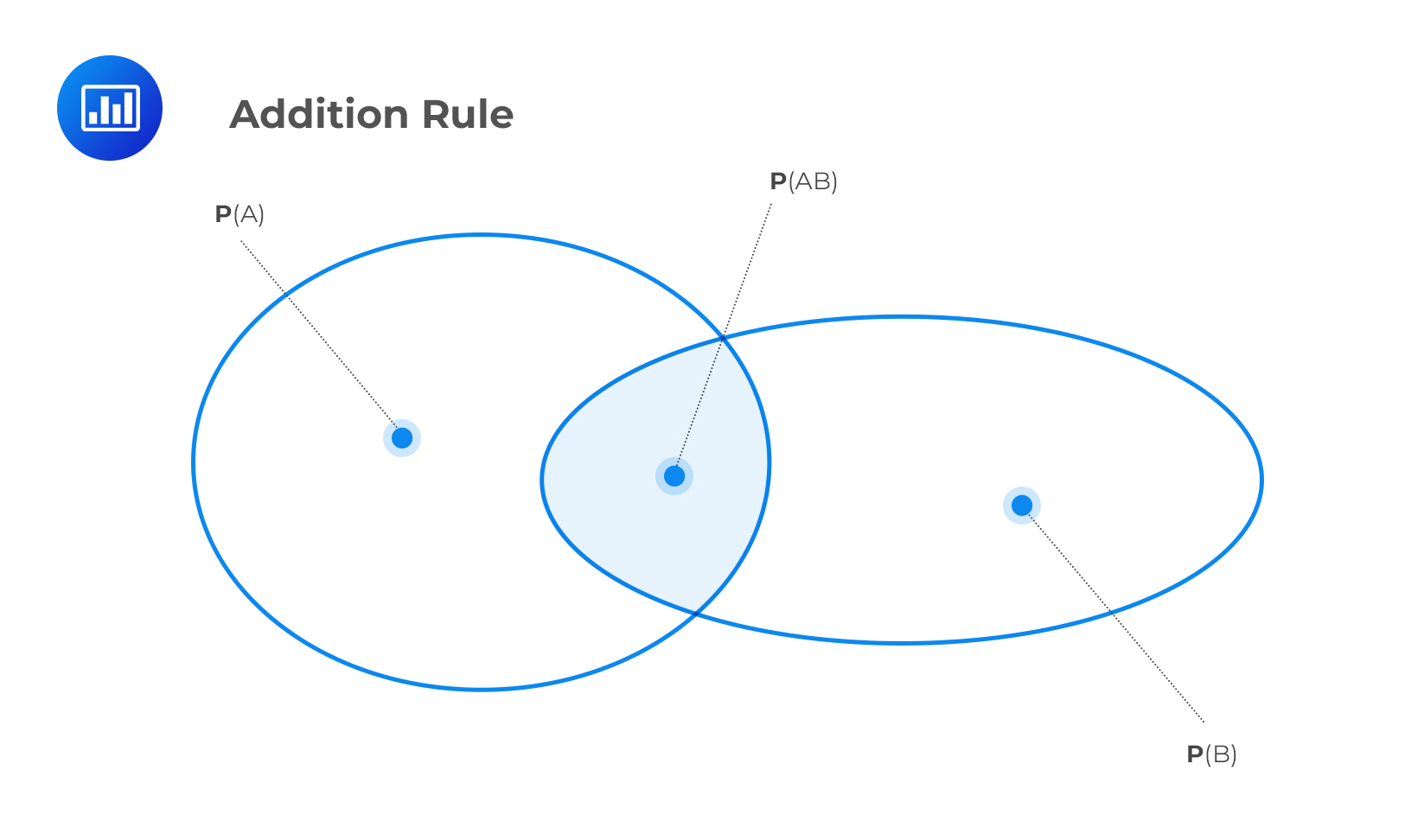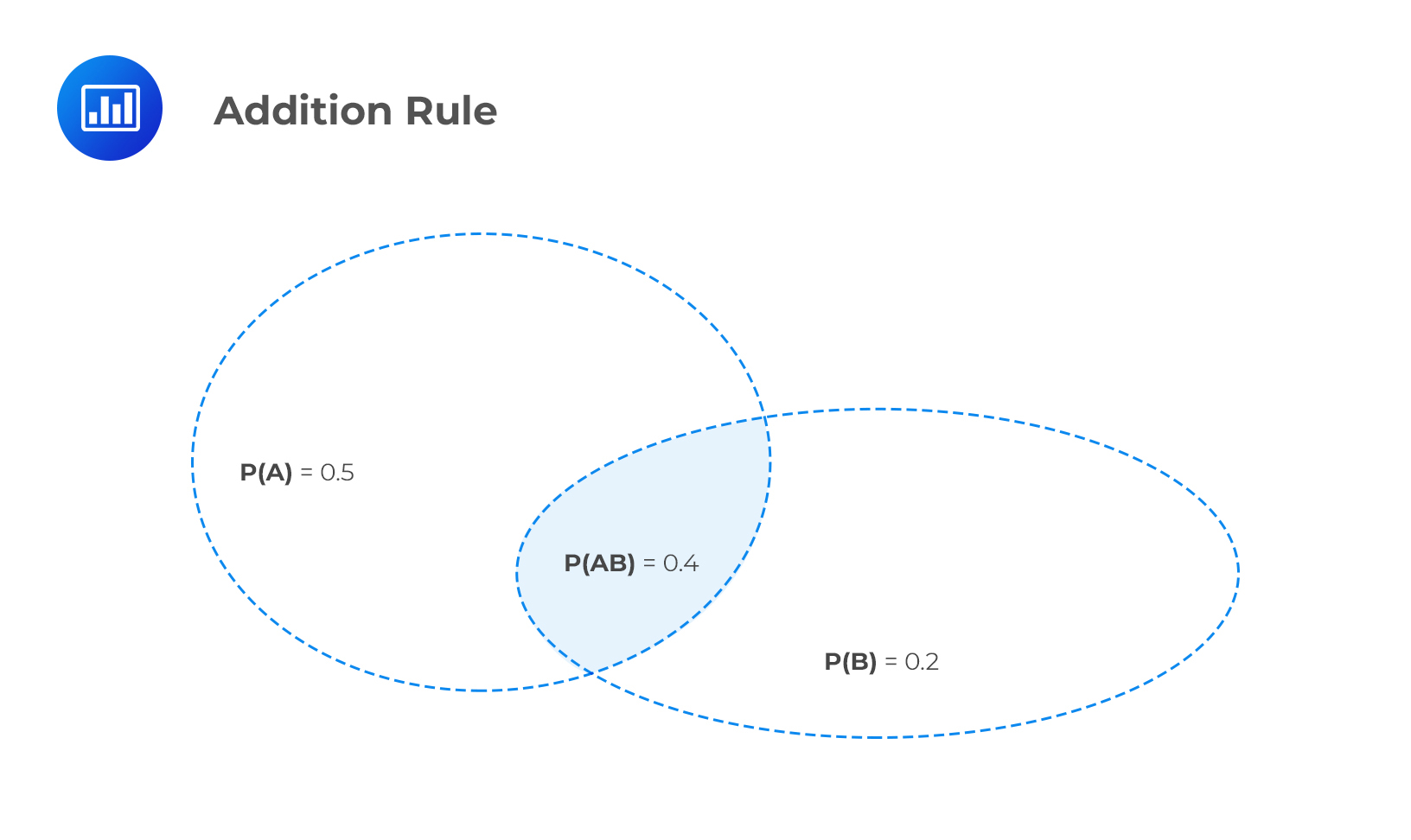Limited Time Offer: Save 10% on all 2022 Premium Study Packages with promo code: BLOG10# Application of Probability Rules

Probability rules are the concepts and facts that must be taken into account while evaluating the probabilities of various events. The CFA curriculum requires candidates to master 3 main rules of probability. These are the multiplication rule, the addition rule, and the law of total probability. We now look at each rule in detail.

## Multiplication Rule

We use the multiplication rule to determine the joint probability of two events, $$P(AB)$$. A joint probability is the probability of two events happening together. For example, we may be interested in the probability that both gas prices and bus fares increase.

### Mutually Exclusive Events

If two events are mutually exclusive, then they cannot occur together. Therefore, we say that such events have zero joint probability.

### Non-Mutually Exclusive Events

For non-mutually exclusive events, the multiplication rule states that:

$$P(AB) = P(A | B)P(B)$$

#### Example: Multiplication Rule

Let’s assume we have a bag containing 16 blue balls and 14 yellow balls. Suppose we draw two balls at random, one after the other without replacement. Let’s define:

Event B = The first ball is blue.

Event A = The second ball is blue.

What will be the joint probability of A and B?

Solution

From the wording of the question, we can calculate the conditional probability $$P(A|B)$$ as:

The probability that the first ball to be drawn is blue, P(B) = $$\cfrac {16}{30}$$.

The probability that the second ball to be drawn is blue given the first one is also blue, P(A | B) = $$\cfrac {15}{29}$$.

Thus,

\begin{align*} P(AB) & = P(A | B)P(B) \\ & = \cfrac {15}{29} × \cfrac {16}{30} \\ & =\cfrac {240}{870} \\ & =\cfrac {8}{29} \\ \end{align*}

We use the addition rule to assess the probability that events A or B occur.

### Mutually Exclusive Events

If A and B are mutually exclusive events:

$$\text P(\text{A or B}) =\text P(\text A) + \text P(\text B)$$

### Non-Mutually Exclusive Events

If A and B are non-mutually exclusive events:

$$\text P(\text{A or B}) =\text P(\text A) + \text P(\text B) – \text P(\text {AB})$$

This is because we have to remove one of the two instances of the intersection of A and B, as shown in the following figure.#### Example: Addition Rule

Suppose the probability of relaxed import restrictions is 0.5 and the probability of a price war is 0.2. If the joint probability of relaxed import restrictions and a price war is 0.4, what is the probability of relaxed trade restrictions or a price war?

Solution

\begin{align} \text P(\text{A or B}) &= \text P(\text A) + \text P(\text B) – \text P(\text {AB}) \\ & = 0.5 + 0.2 – 0.4 = 0.3 \end{align}## Total Probability Rule

Suppose we have a set of mutually exclusive and exhaustive events B1, B2, B3…Bn.

We can determine the unconditional probability of an event, given the conditional probabilities:

$$\text P(\text A) = \text P(\text A | \text B_1)\text P(\text B_1) + \text P(\text A | \text B_3)\text P(\text B_3) + … + \text P(\text A | \text B_n)\text P(\text B_n)$$

Perhaps an example will help you understand the concept:

#### Example: Total Probability Rule

Suppose a local authority subdivides a forest into three regions, B1, B2, and B3. Assume the area covered by each region is 50km2, 65km2, and 74km2 , respectively. What is the total forest area?

Solution

We simply add the respective areas:

$$50 + 65 + 74 = 189 \text{ km}^2$$

## Question

An analyst analyzed defaults of one hundred and fifty corporate bonds, investment grade and non-investment grade. The following table summarizes the results.

$$\begin{array}{l|c|c|c}\\ {}&\textbf{Bonds Default}&\textbf{Bonds did not}&\textbf{Total}\\ {}&{}&\textbf{default}&{}\\ \hline \text{Non-investment}&60&15&75\\ \text{grade bonds}&{}&{}&{}\\ \hline \text{Investment grade}&10&65&75\\ \text{bonds}&{}&{}&{}\\ \hline \text{Total}&70&80&150\\ \end{array}$$

If one bond from the sample of 150 bonds is selected at random, determine the probability that the bond defaulted given that the bond was non-investment grade is:

1. 46.67%.
2. 80.00%.
3. 81.23%.

Solution

The correct answer is B.

The number of bonds that were defaulted and were non-investment grade were 60. The number of bonds that were non-investment grade were 75.

So, the probability that the bond defaulted given that the bond did not default = 60 / 75 = 0.8 = 80%.

A is incorrect. It represents the probability of bonds defaulted out of total sample of 150 bonds (70 / 150).

C is incorrect. It represents the probability that the bond was investment grade given that the bond did not default. The number of bonds that did not default and were investment grade are 65. The number of bonds that did not default were 80.  So the probability that the bond was investment grade given that the bond did not default = 65 / 80 = 81.23%.

Shop CFA® Exam Prep

Offered by AnalystPrepLevel I
Level II
Level III
All Three Levels
Featured Shop FRM® Exam PrepFRM Part I
FRM Part II
FRM Part I & Part II
Learn with Us

Subscribe to our newsletter and keep up with the latest and greatest tips for success
Shop Actuarial Exams PrepExam P (Probability)
Exam FM (Financial Mathematics)
Exams P & FM
Shop GMAT® Exam PrepComplete CourseSergio Torrico
2021-07-23
Excelente para el FRM 2 Escribo esta revisión en español para los hispanohablantes, soy de Bolivia, y utilicé AnalystPrep para dudas y consultas sobre mi preparación para el FRM nivel 2 (lo tomé una sola vez y aprobé muy bien), siempre tuve un soporte claro, directo y rápido, el material sale rápido cuando hay cambios en el temario de GARP, y los ejercicios y exámenes son muy útiles para practicar.diana
2021-07-17
So helpful. I have been using the videos to prepare for the CFA Level II exam. The videos signpost the reading contents, explain the concepts and provide additional context for specific concepts. The fun light-hearted analogies are also a welcome break to some very dry content. I usually watch the videos before going into more in-depth reading and they are a good way to avoid being overwhelmed by the sheer volume of content when you look at the readings.Kriti Dhawan
2021-07-16
A great curriculum provider. James sir explains the concept so well that rather than memorising it, you tend to intuitively understand and absorb them. Thank you ! Grateful I saw this at the right time for my CFA prep.nikhil kumar
2021-06-28
Very well explained and gives a great insight about topics in a very short time. Glad to have found Professor Forjan's lectures.Marwan
2021-06-22
Great support throughout the course by the team, did not feel neglectedBenjamin anonymous
2021-05-10
I loved using AnalystPrep for FRM. QBank is huge, videos are great. Would recommend to a friendDaniel Glyn
2021-03-24
I have finished my FRM1 thanks to AnalystPrep. And now using AnalystPrep for my FRM2 preparation. Professor Forjan is brilliant. He gives such good explanations and analogies. And more than anything makes learning fun. A big thank you to Analystprep and Professor Forjan. 5 stars all the way!michael walshe
2021-03-18
Professor James' videos are excellent for understanding the underlying theories behind financial engineering / financial analysis. The AnalystPrep videos were better than any of the others that I searched through on YouTube for providing a clear explanation of some concepts, such as Portfolio theory, CAPM, and Arbitrage Pricing theory. Watching these cleared up many of the unclarities I had in my head. Highly recommended.